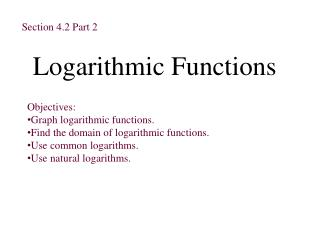DownloadDownload PresentationLogarithmic Functions

# Logarithmic Functions

Télécharger la présentation## Logarithmic Functions

- - - - - - - - - - - - - - - - - - - - - - - - - - - E N D - - - - - - - - - - - - - - - - - - - - - - - - - - -
##### Presentation Transcript

1. Section 4.2 Part 2 Logarithmic Functions • Objectives: • Graph logarithmic functions. • Find the domain of logarithmic functions. • Use common logarithms. • Use natural logarithms.

2. Text Example x -2 -1 0 1 2 3 x 1/4 1/2 1 2 4 8 f (x) = 2x 1/4 1/2 1 2 4 8 g(x) = log2 x -2 -1 0 1 2 3 y = x f (x) = 2x 6 5 4 3 f (x) = log2x 2 -2 -1 2 3 4 5 6 -1 -2 Graph f (x) = 2x and g(x) = log2x in the same rectangular coordinate system. Solution We first set up a table of coordinates for f (x) = 2x. Reversing these coordinates gives the coordinates for the inverse function, g(x) = log2x. Reverse coordinates. We now plot the ordered pairs in both tables, connecting them with smooth curves. The graph of the inverse can also be drawn by reflecting the graph of f (x) = 2x over the line y = x.

3. Characteristics of the Graphs of Logarithmic Functions of the Form f(x) = logbx • The x-intercept is 1. There is no y-intercept. • The y-axis is a vertical asymptote. (x = 0) • If b > 1, the function is increasing. • If 0 < b < 1, the function is decreasing. • The graph is smooth and continuous. It has no sharp corners or edges.

4. General PropertiesCommon Logarithms 1. logb 1 = 0 1. log 1 = 0 2. logbb = 1 2. log 10 = 1 3. logbbx = x 3. log 10x = x 4. b logb x = x 4. 10 log x = x Properties of Common Logarithms

5. log bb = 1 logb 1 = 0 log 4 4 = 1 log 8 1 = 0 3 log 3 6 = 6 log 5 5 3 = 3 2 log 2 7 = 7 Examples of Logarithmic Properties

6. General PropertiesNatural Logarithms 1. logb 1 = 0 1. ln 1 = 0 2. logbb = 1 2. ln e = 1 3. logbbx = x 3. ln ex = x 4. b logb x = x 4. eln x = x Properties of Natural Logarithms

7. log ee = 1 loge 1 = 0 elog e 6 = 6 log ee3 = 3 Examples of Natural Logarithmic Properties